# Creation of Machine Learning Model (Logistic Regression)

## What is this notebook?

This notebook has been made in parallel to Isaac González Machine learning challenge course that you can find in this link ML Challenge.

This course is highly recommended both for people who are just starting out and for people who know but have not touched R and want to see a complete flow with developed in R with the RStudio IDE. It has helped me to know what differences are between R and Python, and above all for adding to my knowledge a brushstroke of the methodology that a professional follows when facing an ML problem.

I have been following the course by using Python language and seeing its parallel capabilities, since all the scripts, more or less, have a 'translation' to python from R

I leave you therefore, if someone gives with this link a summary of the 3 classes of Isaac González developed in python instead of R, for completeness. Fervently I encourage to take his course and follow his classes (approx. 3h) of pure learning.

### Course objective

The objective is to analyze a data set of a machine that can fail suddenly, it has a whole series of instruments attached to it to monitor temperature, humidity and different measurements, we will analyze raw data and finally modeling it which will allow with input data to know if the machine can fail or not, or what is the possibility of failure.

Those of you who follow Isaac González's course: You will see that I try to avoid as much as possible 'spoilers' of the course, but I have to explain something so that the notebook makes some sense, you will also see that it has some additions because I found it interesting.

## Importing Libraries

import pandas as pd
import matplotlib.pyplot as plt
from matplotlib.ticker import PercentFormatter
import seaborn as sns
import numpy as np
%matplotlib inline


## Reading the data and first visualization

df = pd.read_csv("DataSetFallosMaquina.csv",sep=';')

Temperature Humidity Operator Measure1 Measure2 Failure
0 67 82 Operator1 291 1 No
1 68 77 Operator1 1180 1 Yes

## Data Analysis

### Basic Statistics

df.describe()

Temperature Humidity Measure1
count 8784.000000 8784.000000 8784.000000
mean 64.026412 83.337090 1090.900387
std 2.868833 4.836256 537.097769
min 5.000000 65.000000 155.000000
25% 62.000000 80.000000 629.000000
50% 64.000000 83.000000 1096.000000
75% 66.000000 87.000000 1555.000000
max 78.000000 122.000000 2011.000000

Check if there is some null values

df.info()

<class 'pandas.core.frame.DataFrame'>
RangeIndex: 8784 entries, 0 to 8783
Data columns (total 20 columns):
#   Column                        Non-Null Count  Dtype
---  ------                        --------------  -----
0   Temperature                   8784 non-null   int64
1   Humidity                      8784 non-null   int64
2   Operator                      8784 non-null   object
3   Measure1                      8784 non-null   int64
4   Measure2                      8784 non-null   int64
5   Measure3                      8784 non-null   int64
6   Measure4                      8784 non-null   int64
7   Measure5                      8784 non-null   int64
8   Measure6                      8784 non-null   int64
9   Measure7                      8784 non-null   int64
10  Measure8                      8784 non-null   int64
11  Measure9                      8784 non-null   int64
12  Measure10                     8784 non-null   int64
13  Measure11                     8784 non-null   int64
14  Measure12                     8784 non-null   int64
15  Measure13                     8784 non-null   int64
16  Measure14                     8784 non-null   int64
17  Measure15                     8784 non-null   int64
18  Hours Since Previous Failure  8784 non-null   int64
19  Failure                       8784 non-null   object
dtypes: int64(18), object(2)
memory usage: 1.3+ MB


We will see features distribution

df.hist(figsize=(15,15))
plt.show()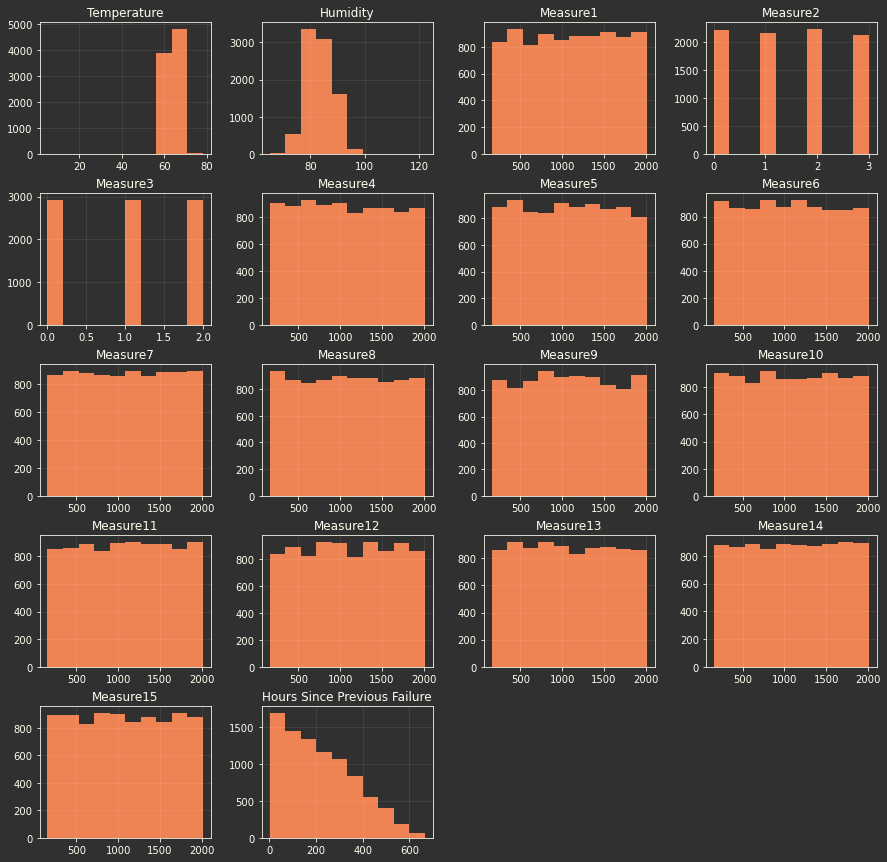We will check if there is some correlation between variables, to delete one of the correlated if it's necessary in our case not, as you could see:

plt.figure(figsize=(10,10))
sns.heatmap(df.corr(method='pearson'))
plt.title('Correlation Matrix')
plt.show()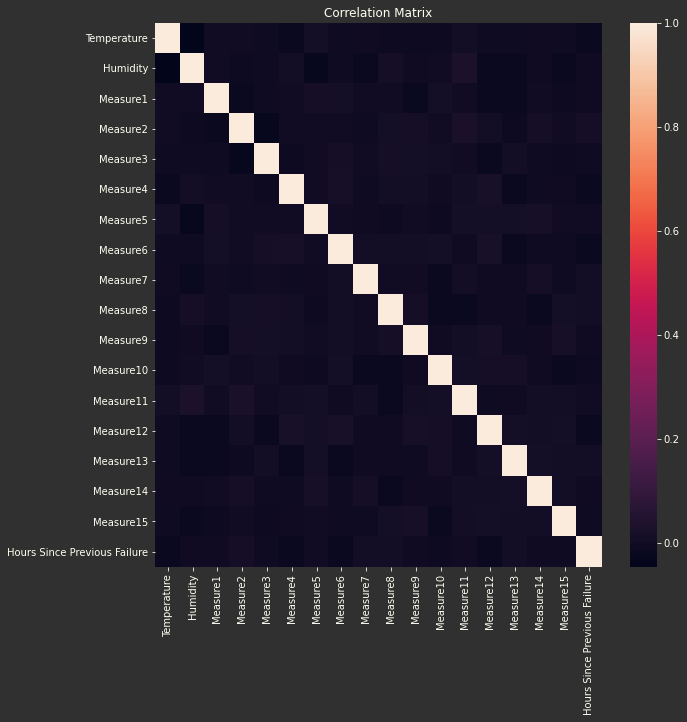More visual elements that can lead us to understand the behavior of variables, their distribution functions, outliers, whether or not they are continuous or categorical variables, etc ...

for col in df:
if df[col].dtype == 'int64':
df[col].plot(kind='kde',figsize=(10,5),title=col,grid=True)
plt.show()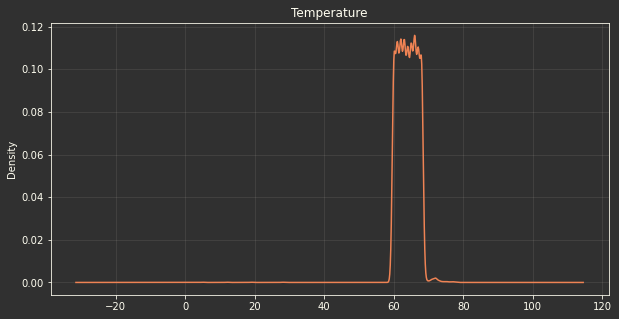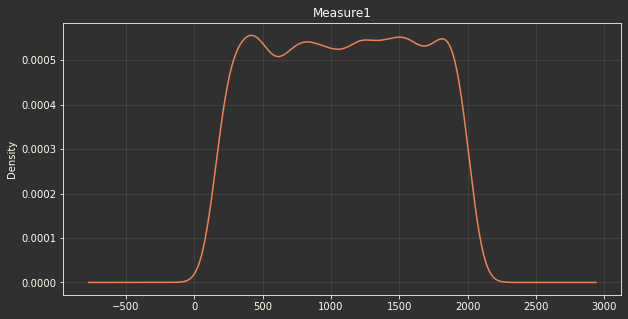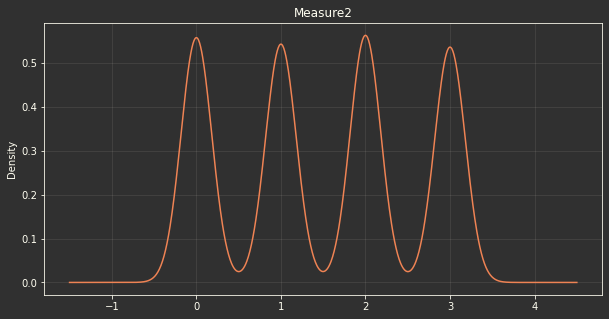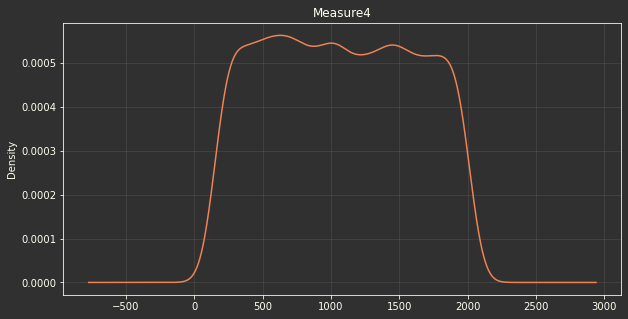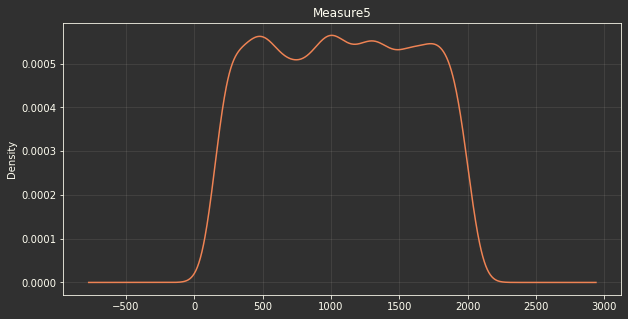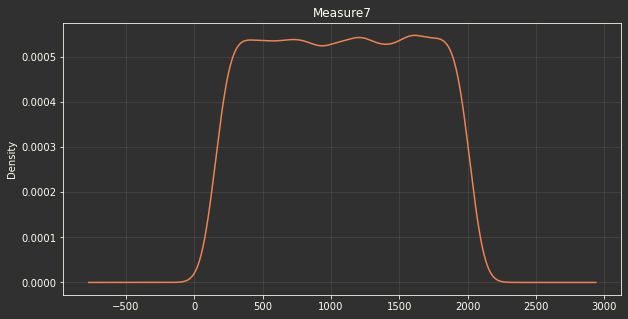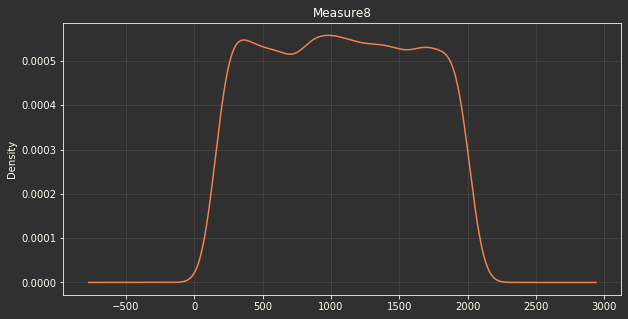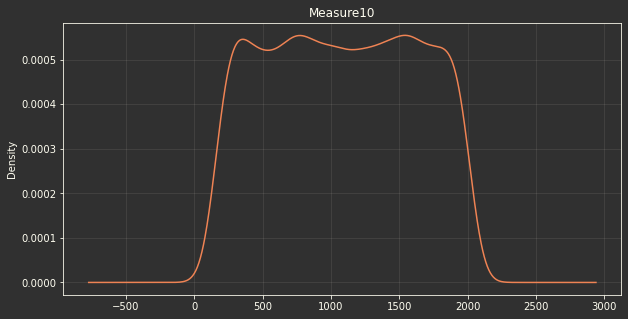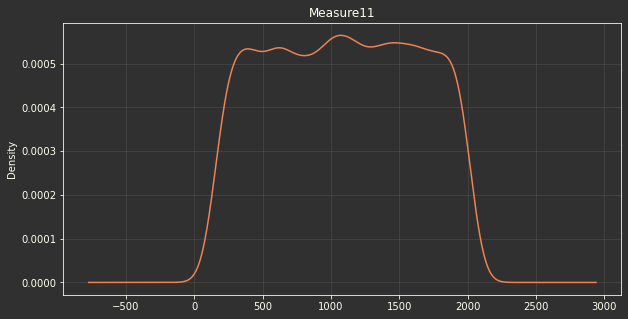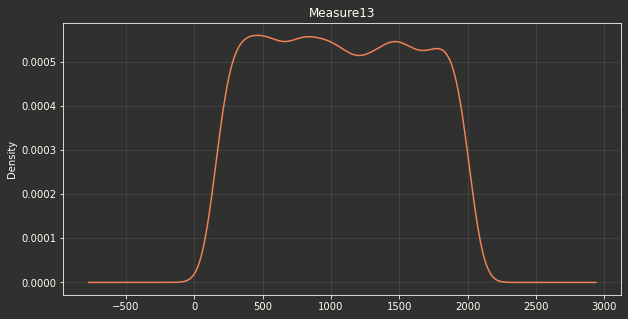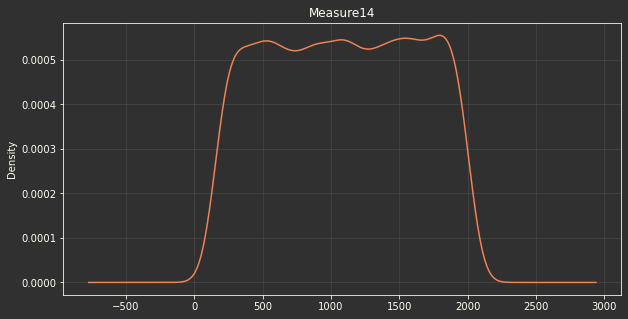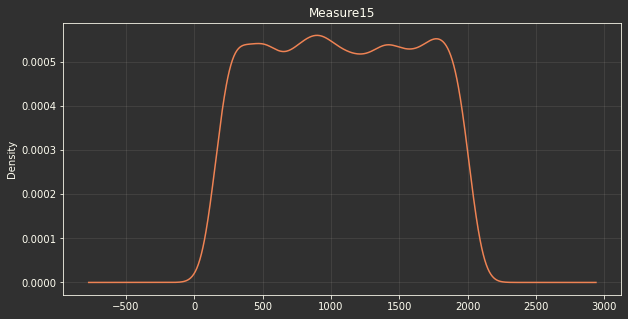for col in df:
if df[col].dtype == 'int64':
df.boxplot(column=col)
plt.show()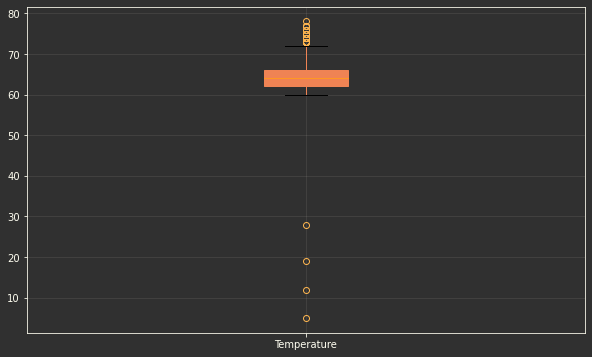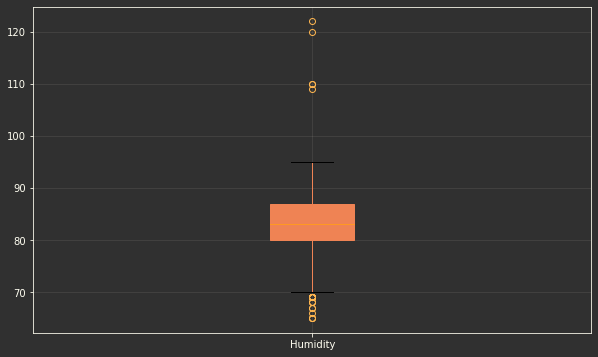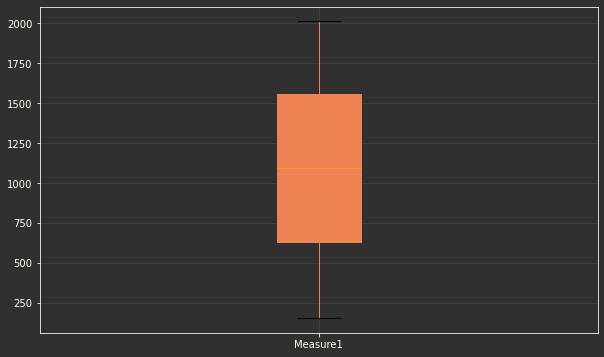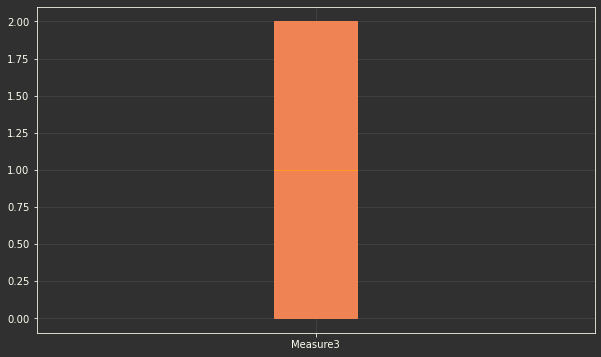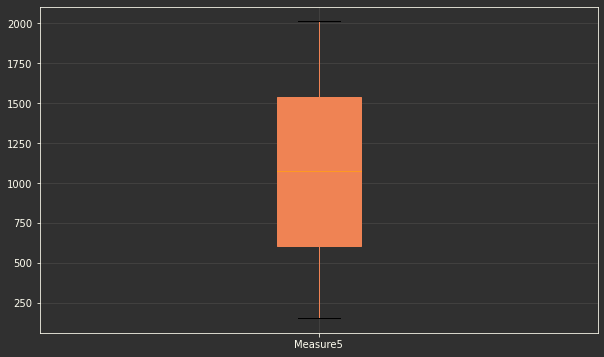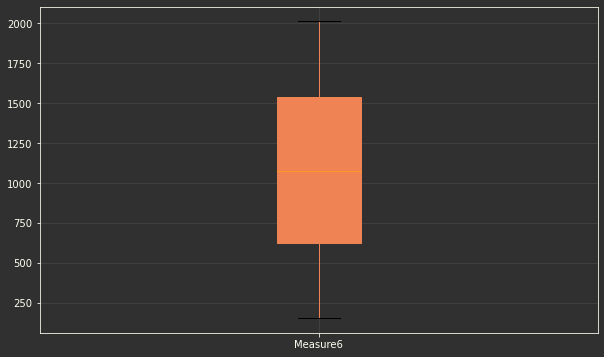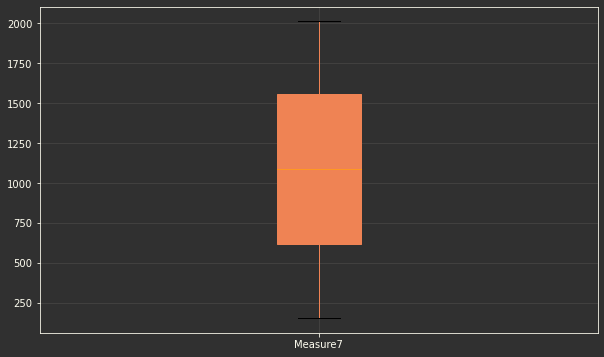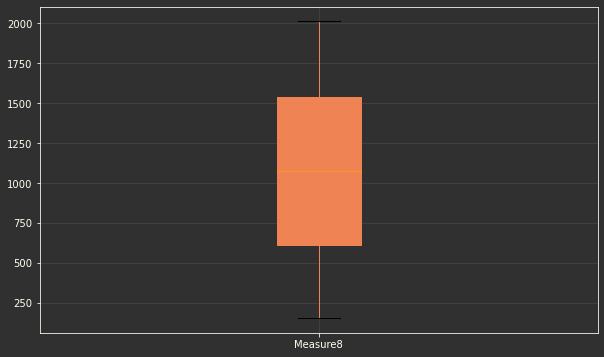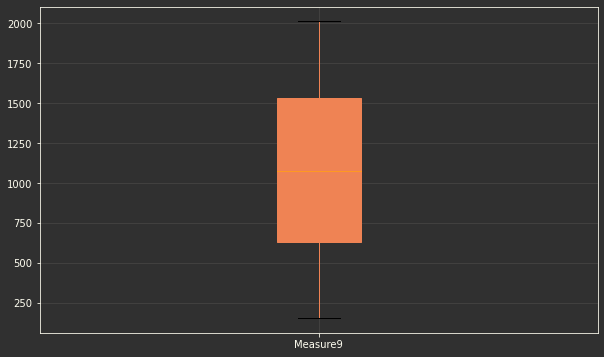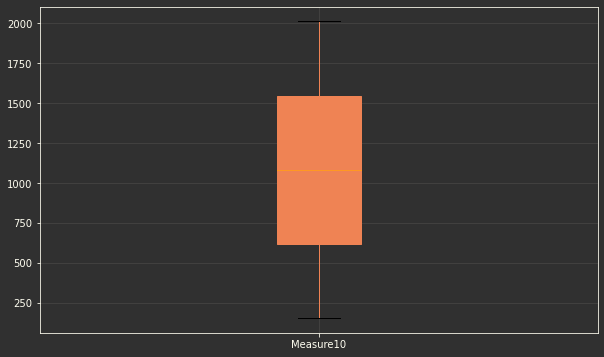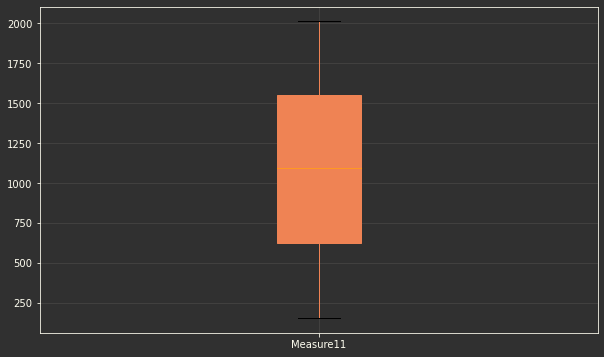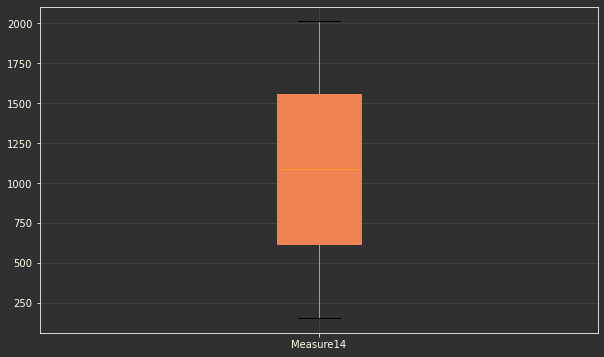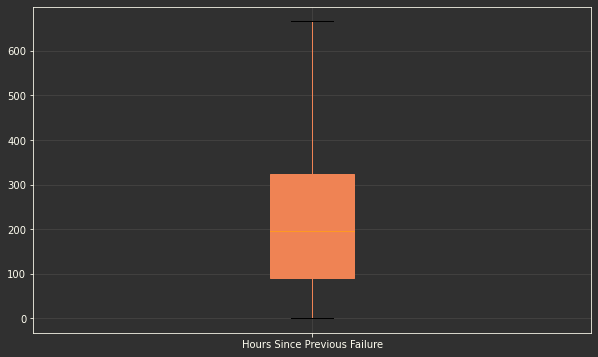### Data Transformation

#### Outliers

df.Temperature.plot(kind='kde')
plt.show()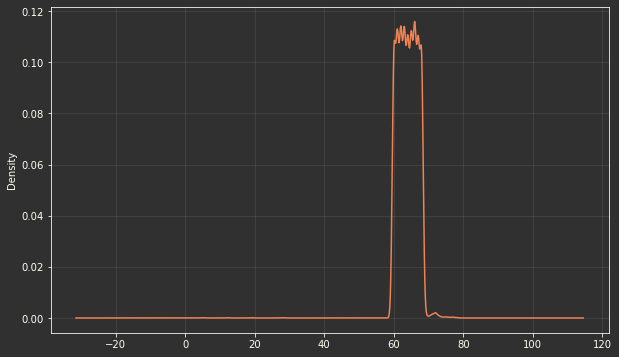sns.boxplot(y='Temperature',data=df)
plt.title('Distribución Temperatura')
plt.show()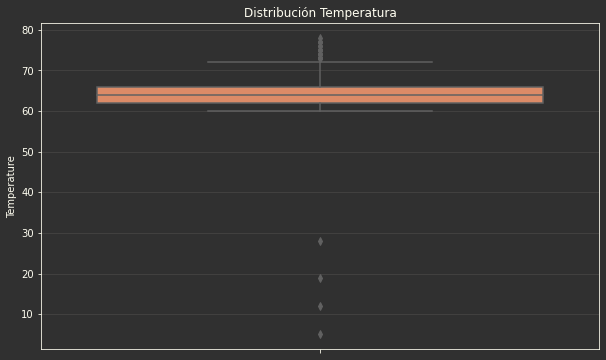df = df[df.Temperature > 50]#drop outliers

df.Temperature.plot(kind='kde')
plt.show()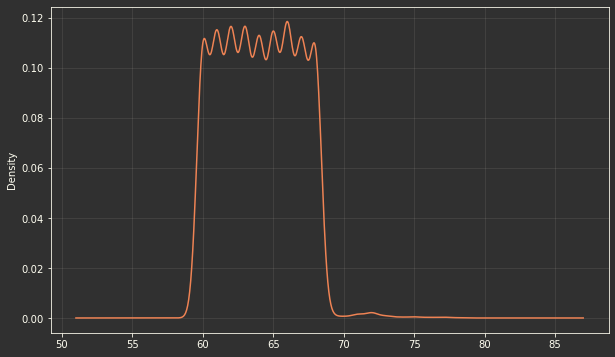sns.boxplot(y='Temperature',data=df)
plt.title('Distribución Temperatura')
plt.show()### Number categorical values to strings

df['Measure2'] = df.Measure2.astype(str)
df['Measure3'] = df.Measure3.astype(str)

df.Measure2.hist()
df.Measure3.hist()
plt.title('Categorical values distribution')
plt.show()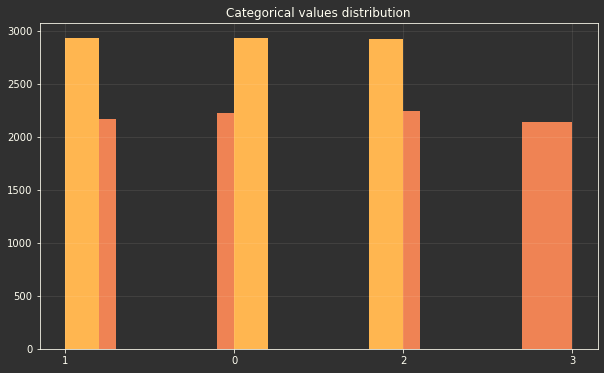### Balancing Data

print('Yes = ','{:.2%}'.format(df['Failure'][df.Failure=='Yes'].count() / df.Failure.count()))
print('No = ','{:.2%}'.format(df['Failure'][df.Failure=='No'].count() / df.Failure.count()))

Yes =  0.92%
No =  99.08%

df_no = df[df.Failure=='No'].sample(frac=0.05,random_state=1234)
df_si = df[df.Failure=='Yes']

df_res = df_no.append(df_si)

print('Yes = ','{:.2%}'.format(df_res['Failure'][df_res.Failure=='Yes'].count() / df_res.Failure.count()))
print('No = ','{:.2%}'.format(df_res['Failure'][df_res.Failure=='No'].count() / df_res.Failure.count()))

Yes =  15.70%
No =  84.30%


### Converting Yes/No values to 1/0 values

df_res.Failure = df_res.Failure.map({'Yes':1,'No':0})

#BACKUP AND CONTINUE
df_backup = df.copy()
df = df_res.copy()


### One-Hot encoder for categorical values

#Operators
df_Operators = pd.get_dummies(df.Operator)
df.drop('Operator',axis=1,inplace=True)

#Measure 2
df_ms2 = pd.get_dummies(df.Measure2)
for col in df_ms2:
new_name = 'Measure2_'+ str(col)
df_ms2.rename(index=str,columns={col: new_name},inplace=True)
df.drop('Measure2',axis=1,inplace=True)

#Measure 3
df_ms3 = pd.get_dummies(df.Measure3)
for col in df_ms3:
new_name = 'Measure3_'+ str(col)
df_ms3.rename(index=str,columns={col: new_name},inplace=True)
df.drop('Measure3',axis=1,inplace=True)

#Concatenate
df.reset_index(drop=True, inplace=True) #reset index for succesful concat.
df_Operators.reset_index(drop=True, inplace=True)
df_ms2.reset_index(drop=True, inplace=True)
df_ms3.reset_index(drop=True, inplace=True)
df_con = pd.concat([df,df_Operators,df_ms2,df_ms3],axis=1)


## Modeling Logistic Regression

### Split in test and training data

from sklearn.linear_model import LogisticRegression
from sklearn.model_selection import train_test_split
from sklearn.metrics import classification_report
from sklearn.metrics import confusion_matrix
from sklearn.metrics import accuracy_score

X = df.drop('Failure',axis=1)
Y = df['Failure']
X_train, X_test, y_train, y_test = train_test_split(X,Y,test_size=0.3)


### Model and fitting data

logmodel = LogisticRegression()
logmodel.fit(X_train, y_train)
print("Logistic Regression",logmodel.get_params())

Logistic Regression {'C': 1.0, 'class_weight': None, 'dual': False, 'fit_intercept': True,
'intercept_scaling': 1, 'l1_ratio': None, 'max_iter': 100, 'multi_class': 'auto',
'n_jobs': None, 'penalty': 'l2', 'random_state': None, 'solver': 'lbfgs',
'tol': 0.0001, 'verbose': 0, 'warm_start': False}


### Accuracy

predictions = logmodel.predict(X_test)

print(classification_report(y_test,predictions))

              precision    recall  f1-score   support

0       0.96      0.96      0.96       135
1       0.75      0.75      0.75        20

accuracy                           0.94       155
macro avg       0.86      0.86      0.86       155
weighted avg       0.94      0.94      0.94       155

conf_matr = confusion_matrix(y_test,predictions)
print(conf_matr)
sns.heatmap(conf_matr,annot=True, cmap="Oranges" ,fmt='g')
plt.tight_layout()
plt.title('Confusion matrix')
plt.ylabel('Actual label')
plt.xlabel('Predicted label')
plt.show()

[[130   5]
[  5  15]]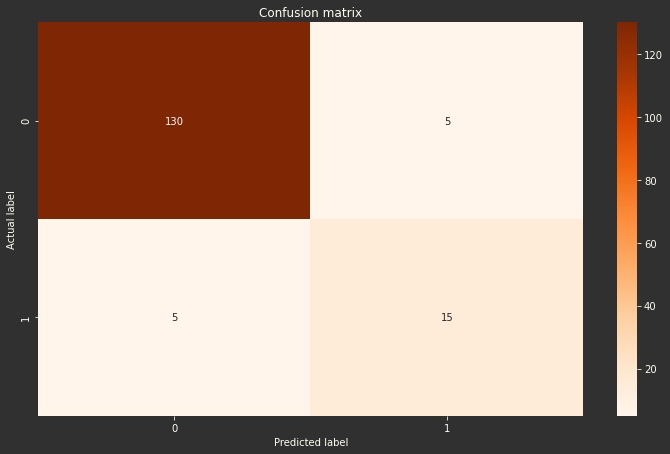print('Accuracy = ','{:.2%}'.format(accuracy_score(y_test,predictions)))

Accuracy =  93.55%

#python #RtoPython #dLogisticRegression

Library
pandas
matplotlib
seaborn
sklearn

Data Origin
csv

### DS Algorithm/Model

Model
Logistic Regression

### Outputs

Function parameters
Accuracy Values